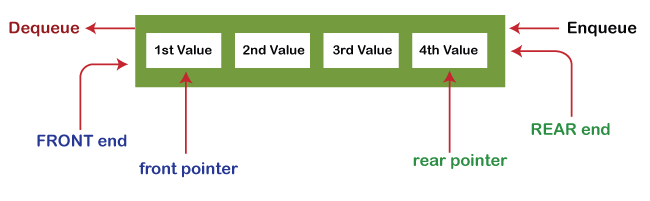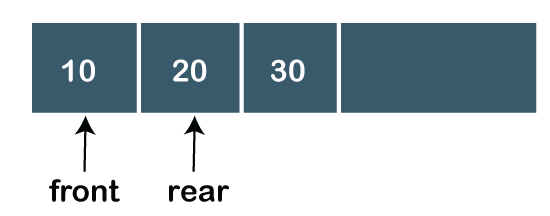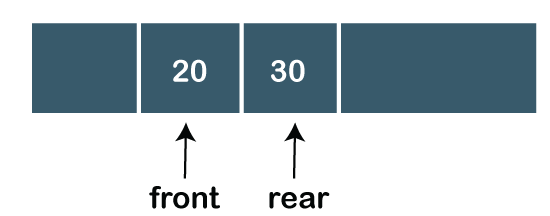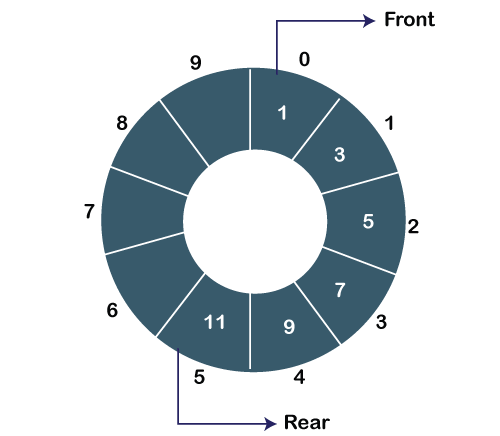# Types of Queues

Before understanding the types of queues, we first look at ’ what is Queue’ .

### What is the Queue?

A queue in the data structure can be considered similar to the queue in the real-world. A queue is a data structure in which whatever comes first will go out first. It follows the FIFO (First-In-First-Out) policy. In Queue, the insertion is done from one end known as the rear end or the tail of the queue, whereas the deletion is done from another end known as the front end or the head of the queue. In other words, it can be defined as a list or a collection with a constraint that the insertion can be performed at one end called as the rear end or tail of the queue and deletion is performed on another end called as the front end or the head of the queue.### Operations on Queue

There are two fundamental operations performed on a Queue:

• Enqueue: The enqueue operation is used to insert the element at the rear end of the queue. It returns void.
• Dequeue: The dequeue operation performs the deletion from the front-end of the queue. It also returns the element which has been removed from the front-end. It returns an integer value. The dequeue operation can also be designed to void.
• Peek: This is the third operation that returns the element, which is pointed by the front pointer in the queue but does not delete it.
• Queue overflow (isfull): When the Queue is completely full, then it shows the overflow condition.
• Queue underflow (isempty): When the Queue is empty, i.e., no elements are in the Queue then it throws the underflow condition.

A Queue can be represented as a container opened from both the sides in which the element can be enqueued from one side and dequeued from another side as shown in the below figure:

### Implementation of Queue

There are two ways of implementing the Queue:

• Sequential allocation: The sequential allocation in a Queue can be implemented using an array.

• Linked list allocation: The linked list allocation in a Queue can be implemented using a linked list.

### What are the use cases of Queue?

Here, we will see the real-world scenarios where we can use the Queue data structure. The Queue data structure is mainly used where there is a shared resource that has to serve the multiple requests but can serve a single request at a time. In such cases, we need to use the Queue data structure for queuing up the requests. The request that arrives first in the queue will be served first. The following are the real-world scenarios in which the Queue concept is used:

• Suppose we have a printer shared between various machines in a network, and any machine or computer in a network can send a print request to the printer. But, the printer can serve a single request at a time, i.e., a printer can print a single document at a time. When any print request comes from the network, and if the printer is busy, the printer’s program will put the print request in a queue.
• . If the requests are available in the Queue, the printer takes a request from the front of the queue, and serves it.
• The processor in a computer is also used as a shared resource. There are multiple requests that the processor must execute, but the processor can serve a single request or execute a single process at a time. Therefore, the processes are kept in a Queue for execution.

### Types of Queue

There are four types of Queues:

• Linear Queue

In Linear Queue, an insertion takes place from one end while the deletion occurs from another end. The end at which the insertion takes place is known as the rear end, and the end at which the deletion takes place is known as front end. It strictly follows the FIFO rule. The linear Queue can be represented, as shown in the below figure:The above figure shows that the elements are inserted from the rear end, and if we insert more elements in a Queue, then the rear value gets incremented on every insertion. If we want to show the deletion, then it can be represented as:In the above figure, we can observe that the front pointer points to the next element, and the element which was previously pointed by the front pointer was deleted.

The major drawback of using a linear Queue is that insertion is done only from the rear end. If the first three elements are deleted from the Queue, we cannot insert more elements even though the space is available in a Linear Queue. In this case, the linear Queue shows the overflow condition as the rear is pointing to the last element of the Queue.

• Circular Queue

In Circular Queue, all the nodes are represented as circular. It is similar to the linear Queue except that the last element of the queue is connected to the first element. It is also known as Ring Buffer as all the ends are connected to another end. The circular queue can be represented as:The drawback that occurs in a linear queue is overcome by using the circular queue. If the empty space is available in a circular queue, the new element can be added in an empty space by simply incrementing the value of rear.

• Priority Queue

A priority queue is another special type of Queue data structure in which each element has some priority associated with it. Based on the priority of the element, the elements are arranged in a priority queue. If the elements occur with the same priority, then they are served according to the FIFO principle.

In priority Queue, the insertion takes place based on the arrival while the deletion occurs based on the priority. The priority Queue can be shown as:

The above figure shows that the highest priority element comes first and the elements of the same priority are arranged based on FIFO structure.

• Deque

Both the Linear Queue and Deque are different as the linear queue follows the FIFO principle whereas, deque does not follow the FIFO principle. In Deque, the insertion and deletion can occur from both ends.